Multivariable Calculus : Partial Differentiation

Example Questions

Example Question #1 : Differentiation Rules

Find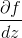.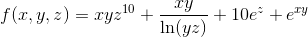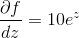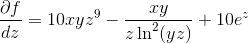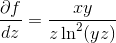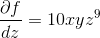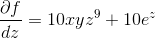Explanation:

In order to find, we need to take the derivative ofin respect to, and treat, andas constants. We also need to remember what the derivatives of natural log, exponential functions and power functions are for single variables.

Natural Log: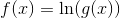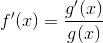Exponential Functions: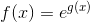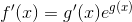Power Functions: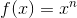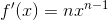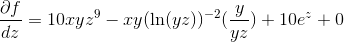Example Question #2 : Differentiation Rules

Find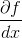.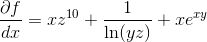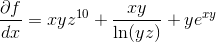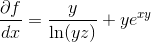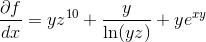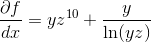Explanation:

In order to find, we need to take the derivative ofin respect to, and treat, andas constants. We also need to remember what the derivatives of natural log, exponential functions and power functions are for single variables.

Natural Log:Exponential Functions:Power Functions: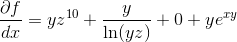Example Question #3 : Differentiation Rules

Find.Explanation:

In order to find, we need to take the derivative ofin respect to, and treat, andas constants. We also need to remember what the derivatives of natural log, exponential functions and power functions are for single variables.

Natural Log:Exponential Functions:Power Functions:Example Question #1 : Arc Length

Determine the length of the curve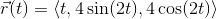, on the interval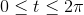.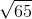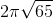Explanation:

First we need to find the tangent vector, and find its magnitude.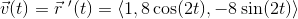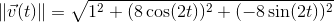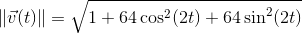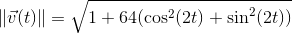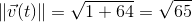Now we can set up our arc length integral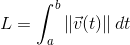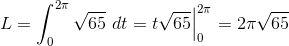Example Question #2 : Partial Differentiation

Determine the length of the curve, on the interval.Explanation:

First we need to find the tangent vector, and find its magnitude.Now we can set up our arc length integral